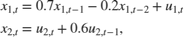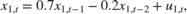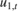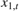# Estimate Time-Varying State-Space Model

This example shows how to create and estimate a state-space model containing time-varying parameters.

Suppose that an AR(2) and an MA(1) model comprise a latent process. There are 50 periods, and the MA(1) process drops out of the model for the final 25 periods. The state equation for the first 25 periods isFor the last 25 periods, the state equation iswhereandare Gaussian with mean 0 and standard deviation 1.

Generate a random series of 50 observations fromand, assuming that the series starts at 1.5 and 1, respectively.

T = 50; ARMdl = arima('AR',{0.7,-0.2},'Constant',0,'Variance',1); MAMdl = arima('MA',0.6,'Constant',0,'Variance',1); x0 = [1.5 1; 1.5 1]; rng(1); x = [simulate(ARMdl,T,'Y0',x0(:,1)),... [simulate(MAMdl,T/2,'Y0',x0(:,2));nan(T/2,1)]]; 

The last 25 values for the simulated MA(1) data are missing.

Suppose further that the latent processes are measured usingfor the first 25 periods, andfor the last 25 periods.is Gaussian with mean 0 and standard deviation 1.

Generate observations using the random latent state process (x) and the observation equation.

y = 2*sum(x','omitnan')'+randn(T,1); 

Together, the latent process and observation equations compose a state-space model. Supposing that the coefficients are unknown parameters, the state-space model isWrite a function that specifies how the parameters in params map to the state-space model matrices, the initial state values, and the type of state.

 % Copyright 2015 The MathWorks, Inc. function [A,B,C,D,Mean0,Cov0,StateType] = AR2MAParamMap(params,T) %AR2MAParamMap Time-variant state-space model parameter mapping function % % This function maps the vector params to the state-space matrices (A, B, % C, and D), the initial state value and the initial state variance (Mean0 % and Cov0), and the type of state (StateType). From periods 1 to T/2, the % state model is an AR(2) and an MA(1) model, and the observation model is % the sum of the two states. From periods T/2 + 1 to T, the state model is % just the AR(2) model. A1 = {[params(1) params(2) 0 0; 1 0 0 0; 0 0 0 params(3); 0 0 0 0]}; B1 = {[1 0; 0 0; 0 1; 0 1]}; C1 = {params(4)*[1 0 1 0]}; Mean0 = ones(4,1); Cov0 = 10*eye(4); StateType = [0 0 0 0]; A2 = {[params(1) params(2) 0 0; 1 0 0 0]}; B2 = {[1; 0]}; A3 = {[params(1) params(2); 1 0]}; B3 = {[1; 0]}; C3 = {params(5)*[1 0]}; A = [repmat(A1,T/2,1);A2;repmat(A3,(T-2)/2,1)]; B = [repmat(B1,T/2,1);B2;repmat(B3,(T-2)/2,1)]; C = [repmat(C1,T/2,1);repmat(C3,T/2,1)]; D = 1; end 

Save this code in a file named AR2MAParamMap and put it in your MATLAB® path.

Create the state-space model by passing the function AR2MAParamMap as a function handle to ssm.

Mdl = ssm(@(params)AR2MAParamMap(params,T)); 

ssm implicitly defines the state-space model. Usually, you cannot verify implicitly defined state-space models.

Pass the observed responses (y) to estimate to estimate the parameters. Specify positive initial values for the unknown parameters.

params0 = 0.1*ones(5,1); EstMdl = estimate(Mdl,y,params0) 
Method: Maximum likelihood (fminunc) Sample size: 50 Logarithmic likelihood: -114.957 Akaike info criterion: 239.913 Bayesian info criterion: 249.473 | Coeff Std Err t Stat Prob --------------------------------------------------- c(1) | 0.47870 0.26634 1.79733 0.07229 c(2) | 0.00809 0.27179 0.02975 0.97626 c(3) | 0.55735 0.80958 0.68844 0.49118 c(4) | 1.62679 0.41622 3.90848 0.00009 c(5) | 1.90021 0.49563 3.83391 0.00013 | | Final State Std Dev t Stat Prob x(1) | -0.81229 0.46815 -1.73511 0.08272 x(2) | -0.31449 0.45918 -0.68490 0.49341 EstMdl = State-space model type: <a href="matlab: doc ssm">ssm</a> State vector length: Time-varying Observation vector length: 1 State disturbance vector length: Time-varying Observation innovation vector length: 1 Sample size supported by model: 50 State variables: x1, x2,... State disturbances: u1, u2,... Observation series: y1, y2,... Observation innovations: e1, e2,... State equations of period 1, 2, 3,..., 25: x1(t) = (0.48)x1(t-1) + (8.09e-03)x2(t-1) + u1(t) x2(t) = x1(t-1) x3(t) = (0.56)x4(t-1) + u2(t) x4(t) = u2(t) State equations of period 26: x1(t) = (0.48)x1(t-1) + (8.09e-03)x2(t-1) + u1(t) x2(t) = x1(t-1) State equations of period 27, 28, 29,..., 50: x1(t) = (0.48)x1(t-1) + (8.09e-03)x2(t-1) + u1(t) x2(t) = x1(t-1) Observation equation of period 1, 2, 3,..., 25: y1(t) = (1.63)x1(t) + (1.63)x3(t) + e1(t) Observation equation of period 26, 27, 28,..., 50: y1(t) = (1.90)x1(t) + e1(t) Initial state distribution: Initial state means x1 x2 x3 x4 1 1 1 1 Initial state covariance matrix x1 x2 x3 x4 x1 10 0 0 0 x2 0 10 0 0 x3 0 0 10 0 x4 0 0 0 10 State types x1 x2 x3 x4 Stationary Stationary Stationary Stationary 

The estimated parameters are within 1 standard error of their true values, but the standard errors are quite high. Likelihood surfaces of state-space models might contain local maxima. Therefore, it is good practice to try several initial parameter values, or consider using refine.